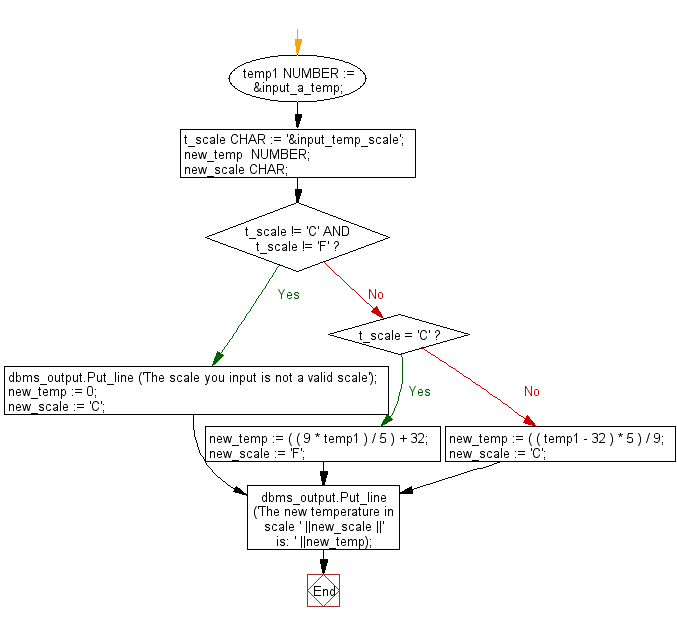﻿ PL/SQL Control Statement: Convert a temperature in scale Fahrenheit to Celsius and vice versa - w3resource# PL/SQL Control Statement Exercises: Convert a temperature in scale Fahrenheit to Celsius and vice versa

## PL/SQL Control Statement: Exercise-14 with Solution

Write a PL/SQL program to convert a temperature in scale Fahrenheit to Celsius and vice versa.

Sample Solution:

PL/SQL Code:

``````DECLARE
temp1     NUMBER := &input_a_temp;
t_scale   CHAR := '&input_temp_scale';
new_temp  NUMBER;
new_scale CHAR;
BEGIN
IF t_scale != 'C'
AND
t_scale != 'F' THEN
dbms_output.Put_line ('The scale you input is not a valid scale');
new_temp := 0;
new_scale := 'C';
ELSE
IF t_scale = 'C' THEN
new_temp := ( ( 9 * temp1 ) / 5 ) + 32;
new_scale := 'F';
ELSE
new_temp := ( ( temp1 - 32 ) * 5 ) / 9;
new_scale := 'C';
END IF;
END IF;
dbms_output.Put_line ('The new temperature in scale '
||new_scale
||' is: '
||new_temp);
END;
/
```
```

Sample Output:

``` SQL> /
Enter value for input_a_temp: 100
old   2:     temp1     NUMBER := &input_a_temp;
new   2:     temp1     NUMBER := 100;
Enter value for input_temp_scale: C
old   3:     t_scale   CHAR := '&input_temp_scale';
new   3:     t_scale   CHAR := 'C';
The new temperature in scale F is: 212

PL/SQL procedure successfully completed.
```

Sample Output:

```SQL> /
Enter value for input_a_temp: 212
old   2:     temp1     NUMBER := &input_a_temp;
new   2:     temp1     NUMBER := 212;
Enter value for input_temp_scale: F
old   3:     t_scale   CHAR := '&input_temp_scale';
new   3:     t_scale   CHAR := 'F';
The new temperature in scale C is: 100

PL/SQL procedure successfully completed.
```

Sample Output:

```SQL> /
Enter value for input_a_temp: 100
old   2:     temp1     NUMBER := &input_a_temp;
new   2:     temp1     NUMBER := 100;
Enter value for input_temp_scale: V
old   3:     t_scale   CHAR := '&input_temp_scale';
new   3:     t_scale   CHAR := 'V';
The scale you input is not a valid scale
The new temperature in scale C is: 0

PL/SQL procedure successfully completed.
```

Flowchart:Improve this sample solution and post your code through Disqus

What is the difficulty level of this exercise?

﻿